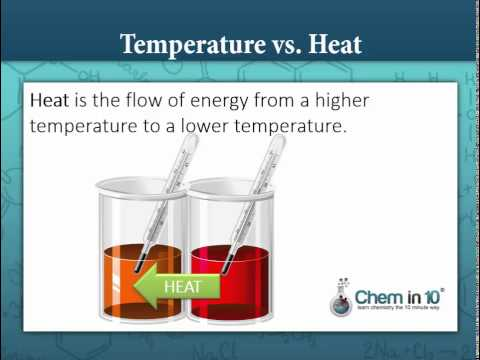### Main Difference

People often use the terms heat and temperature interchangeably without actually understanding between both of them. It should be kept noted that both these concepts are studied in the science, and are interrelated to each other. In other words, we can say that temperature is the subset of the heat; this can be further elaborated by defining both these concepts. Heat is the overall energy, either kinetic or potential energy possessed by the body. On the other hand, the temperature is the measure of the heat intensity present in the body. The transfer of heat energy from the one body to the other takes place due to the difference in temperature of the bodies. It should be kept noted here that heat energy travels from a hotter object to the cooler object.

### Comparison Chart

 Heat Temperature Definition Heat is the total amount of energy i.e. kinetic and potential energy possessed by the body. The temperature is the measure of heat intensity of the body/object. Measure The heat is the measure of both the energy’s within an object, either kinetic or potential. The temperature is the measure of the average kinetic energy possessed by the object. Transfer The heat transmission takes from hotter object to the cooler object. Temperature rises or falls with a change in the heat as it is directly dependent on heat. SI Unit Joules Kelvin Device Calorimeter Thermometer Symbol ‘Q’ ‘T’

### What is Heat?

Heat is the form of energy, which is the measure of the total amount of energy possessed by the object. The heat energy gets higher or lower all because of the movement of smaller particles like atoms, ions or molecules within the body. As we know that the object either solid, liquid or gas comprises of microscopic particles like atoms, molecules or ions; the movement of such particles make up the total heat energy of the body. When we talk about the energy in the case of heat; it is the total energy of the body i.e. kinetic energy and the potential energy. The heat energy gets transferred from one object to the other object due to the difference in the temperatures. The heat always flows from the hotter object to the cooler object. The measurement of heat energy is done in the units like calorie or joules using the calorie meter or any other similar device. The exchange of heat can mainly take place through three ways: conduction, reduction, and radiation. The transmission of heat energy taking place between two bodies without the movement of the material is known as the conduction. On the other hand, the heat energy taking place due to the movement of the subatomic particle is known as the convection.

### What is Temperature?

Temperature is the measure of the heat intensity of the body; in other words, we can say that temperature is the average kinetic energy possessed by the body. It should be noted that the temperature is the subset of the heat and it is directly dependent on the amount of heat. It can be further expected, when the object is heated the temperature of the object increases, and when the object is cooled the temperature decreases. The temperature is the average amount of kinetic energy possessed by the body; it has nothing to do with the size and the type of the body. Other than the average measure of kinetic energy, the temperature also evaluates the speed of the atoms and molecules of the object. The thermometer is the apparatus used to measure the temperature, and it is denoted using the units like Kelvin, Fahrenheit, and Celsius.

### Heat vs. Temperature

• Heat is the total amount of energy i.e. kinetic and potential energy possessed by the body. On the other hand, the temperature is the measure of heat intensity of the body/object.
• The heat is the measure of both the energy’s within an object, either kinetic or potential energy. Contrary to this, the temperature is the measure of the average kinetic energy possessed by the object.
• The heat transmission takes from hotter object to the cooler object, whereas temperature rises or falls with a change in the heat as it is directly dependent on heat.
• The SI unit of heat is Joules while SI unit of temperatures is Kelvin.
• The calorimeter is used to measure the heat of the object, whereas thermometer is used to measure the temperature.
• Heat is denoted using the symbol ‘Q,’ whereas symbol ‘T’ represents the temperature.